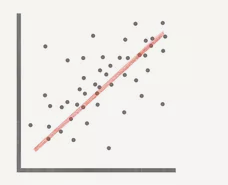Coefficient homework Help at TutorEye

## Coefficient:

A coefficient is an integer multiplied by a single term's variable or the polynomial terms.In an expression,  ax2+bx+c,where x is the variable and a and b are the coefficients.

## Coefficient Sample Questions:

Question 1: Choose the numerical coefficient of the term −8yz?

(a) 8

(b) 8y

(c) -8

(d) -8y

Explanation: -8 is the numerical value and rest y and z are variables.

Question 2: Choose the numerical coefficient of x2 the term  4x2 +6x+9 ?

(a) 4

(b) 6

(c) 9

(d) -4

Explanation: 4 is the cofficient of x2

Question 3: Choose the correct option for the given graph ?(a) Negative correlation

(b) Positive correlation

(c) No-correlation

(d) None

Explanation: It is the graph of positive correlation, as it going in upward direction.

Question 4: Which correlation technique we used for finding correlation coefficients if we are dealing with quantitative characteristics?

(a) Karl Pearson’s Correlation Coefficient

(b) Spearman’s Rank Correlation Coefficient

(c) Both a and b

(d) None

Explanation: Karl Pearson’s correlation coefficient  is used for quantitative data while Spearman’s Rank Correlation Coefficient is used for qualitative data.

Question 5: A 5 kg box on a horizontal table is pushed by a horizontal force. If the coefficient of friction is 0.4, find the horizontal force?

(a) 19.6 N

(b) 2 N

(c) 15 N

(d) 3.92 N

Explanation: We used the formula of friction coefficient

Question 6: A 5 kg box on a horizontal table is pushed by a horizontal force of 25N. Find the coefficient of friction required to move the box from its stationary position?

(a) 0.51

(b) 0.41

(c) 5

(d) 4

Explanation: We used the formula of friction coefficient

Question 7: The mean of the data is 25.5 and its standard deviation is 4.2,find the coefficient of variation?

(a) 16.5%

(b) 15%

(c) 15.5%

(d) None

Explanation: We used the formula of friction coefficient.

Question 8: The mean of the data is 68.5 and its coefficient of variation is 25.75,find the standard deviation of the data?

(a) 16.5

(b) 15

(c) 17.6

(d) None

Explanation: We used the formula of coefficient of variation.

Question 9: Adam loves to collect books, he also gets books as a gift from his dad every year on his birthday. Last year he found 68 books in his bookshelf. So far this year he has collected 18 books. How many books does Adam have in his collection?

(a) 80

(b) 90

(c) 100

(d) 50

Explanation: We used the formula of coefficient of variation.

Question 10: Find the coefficient of x3,x2,and x in equation 5x3+2x2-x+6=0?

(a) 5, -1, 2

(b) -1, 2, 5

(c) 5, 2, -1

(d) 2, -1,5